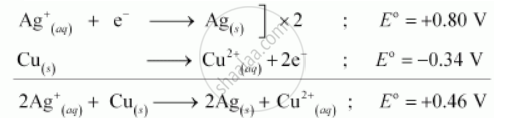Share

# Using the standard electrode potentials given in Table 3.1, predict if the reaction between the following is feasible: Ag+ (aq) and Cu(s) - CBSE (Science) Class 12 - Chemistry

ConceptElectrolytic Cells and Electrolysis Products of Electrolysis

#### Question

Using the standard electrode potentials given in Table 3.1, predict if the reaction between the following is feasible:

Ag+ (aq) and Cu(s)

#### SolutionSince E^@ for the overall reaction is positive, the reaction between Ag+ (aq) and Cu(s) is feasible

Is there an error in this question or solution?

#### APPEARS IN

NCERT Solution for Chemistry Textbook for Class 12 (2018 to Current)
Chapter 3: Electrochemistry
Q: 17.2 | Page no. 92

#### Video TutorialsVIEW ALL 

Solution Using the standard electrode potentials given in Table 3.1, predict if the reaction between the following is feasible: Ag+ (aq) and Cu(s) Concept: Electrolytic Cells and Electrolysis - Products of Electrolysis.
S